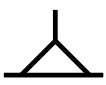Question 8

# Select the figure in which the given figure is embedded.Solution

in this question we have to find out the figure which have the exact figure given in the question .

basically we have to find out the triangle shape figure in given options , we are seeing that triangle is only in the option C so our answer will be option c

• Free SSC Study Material - 18000 Questions
• 230+ SSC previous papers with solutions PDF